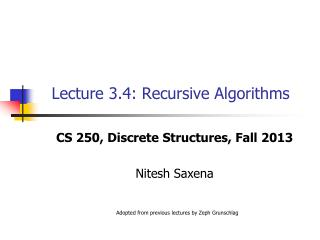# Lecture 3.4: Recursive Algorithms - PowerPoint PPT PresentationDownload PresentationLecture 3.4: Recursive Algorithms

Lecture 3.4: Recursive AlgorithmsDownload Presentation## Lecture 3.4: Recursive Algorithms

- - - - - - - - - - - - - - - - - - - - - - - - - - - E N D - - - - - - - - - - - - - - - - - - - - - - - - - - -
##### Presentation Transcript

1. Lecture 3.4: Recursive Algorithms CS 250, Discrete Structures, Fall 2013 Nitesh Saxena Adopted from previous lectures by Zeph Grunschlag

2. Course Admin • Graded Mid-Term 1 • Please pick them up, if you haven’t done so • Graded HW2 • Please pick them up, if you haven’t done so • HW3 posted • Covers “Induction and Recursion” (Chapter 5) • Due 11am Nov 7 (Thursday)

3. Course Admin • Mid Term 2 • Nov 5 (Tues) • Covers “Induction and Recursion” (Chapter 5) • Review Oct 31 (Thu)

4. Outline • Recursive Algorithms and Programs • Proving the correctness of recursive programs

5. Recursive Algorithms Computer Implementation long factorial(int n){ if (n==0) return 1; return n*factorial(n-1); } Compute 5!

6. Recursive Algorithms Computer Implementation long factorial(int n){ if (n==0) return 1; return n*factorial(n-1); }

7. Recursive Algorithms Computer Implementation long factorial(int n){ if (n==0) return 1; return n*factorial(n-1); }

8. Recursive Algorithms Computer Implementation long factorial(int n){ if (n==0) return 1; return n*factorial(n-1); }

9. Recursive Algorithms Computer Implementation long factorial(int n){ if (n==0) return 1; return n*factorial(n-1); }

10. Recursive Algorithms Computer Implementation long factorial(int n){ if (n==0) return 1; return n*factorial(n-1); }

11. Recursive Algorithms Computer Implementation long factorial(int n){ if (n==0) return 1; return n*factorial(n-1); }

12. Recursive Algorithms Computer Implementation long factorial(int n){ if (n==0) return 1; return n*factorial(n-1); }

13. Recursive Algorithms Computer Implementation long factorial(int n){ if (n==0) return 1; return n*factorial(n-1); }

14. Recursive Algorithms Computer Implementation long factorial(int n){ if (n==0) return 1; return n*factorial(n-1); }

15. Recursive Algorithms Computer Implementation long factorial(int n){ if (n==0) return 1; return n*factorial(n-1); }

16. Recursive Algorithms Computer Implementation long factorial(int n){ if (n==0) return 1; return n*factorial(n-1); }

17. Recursive Algorithms Computer Implementation long factorial(int n){ if (n==0) return 1; return n*factorial(n-1); } Return 5! = 120

18. From Recursive DefinitionsTo Recursive Algorithms In general, starting a recursive function: gives a recursive algorithm: output-type f(input-type n) { if (n in range1) return output1 … if (n in rangek) return outputk }

19. Efficiency of Recursive AlgorithmsExamples We can also turn the recursive definitions of the Fibonacci function, and the gcd into recursive algorithms, but in the case of Fibonacci, these algorithms are really bad (as far as running time is concerned). Here’s the pseudocode for these examples:

20. Recursive AlgorithmsFibonacci integer f (non-neg. integer n){ if (n 1) return n return f (n -1) + f (n -2) } We need to at most 2n calls to the function f() to be able to evaluate it for input n. Because going from n to n-1 spawns off 2 method calls, and each of these, in turn, spawns 2 threads, and so on. Even if n is moderately large, this algorithm will take a long time to compute

21. Recursive AlgorithmsFibonacci Q: Is there a better algorithm? A: Use iterative algorithm instead of a recursive one, especially for large numbers integer fibonacci(non-neg-integer n){ f=0, f=1 //array or sequence for (i = 2 to n ) { f[i] = f[i-1] + f[i-2] } return f[n] }

22. Recursive Algorithmsgcd integer gcd(positive integerx,positive integery) { if (y == 0) return x return gcd(y,x % y) } Running time: The algorithm is actually very efficient. It is linear with respect to the length of the largest input. Note that every time you recursively call the function, the size of inputs reduces.

23. Program Correctness Induction can be used to show that programs using iterative loops are correct. Ex: Prove that the following program correctly calculates Fibonacci sequence: integer fibonacci(non-neg-integer n){ f=0, f=1 for (i = 2 to n ) { f[i] = f[i-1] + f[i-2] } return f[n] }

24. Program CorrectnessFibonacci Example integer fibonacci(non-neg-integer n){ f=0, f=1 for (i = 2 to n ) { f[i] = f[i-1] + f[i-2] } return f[n] } • Apply strong mathematical induction • Show that the program returns the correct output for n=0 • Assume that the program returns the correct output for n=k, n=k-1,…,n=0, and using this assumption, show that the program returns the correct output for n=k+1 • Let’s use the whiteboard to do this proof!

25. Program CorrectnessFibonacci Example integer fibonacci(non-neg-integer n){ for (i = 2 to n ) { f[i] = f[i-1] + f[i-2] } return f[n] } What’s wrong here?

26. Today’s Reading • Rosen 5.4